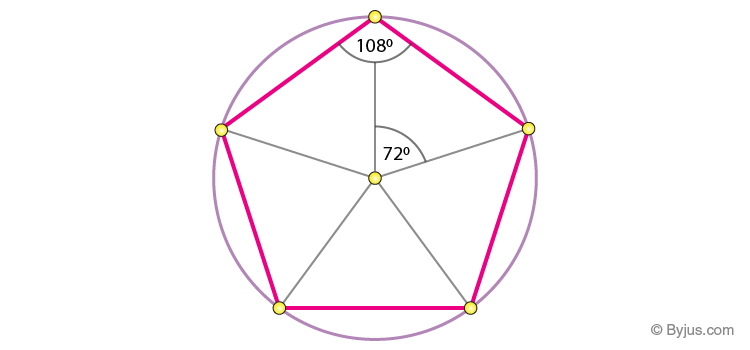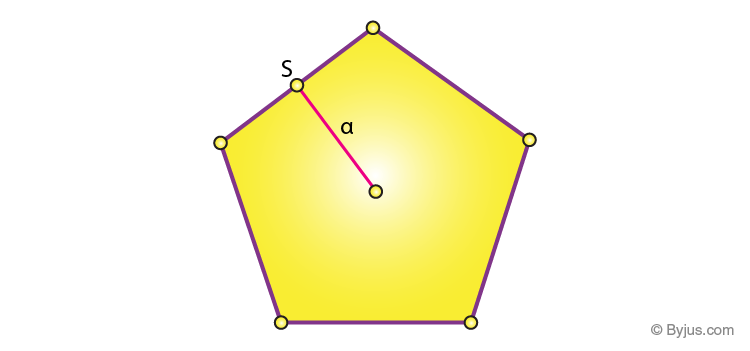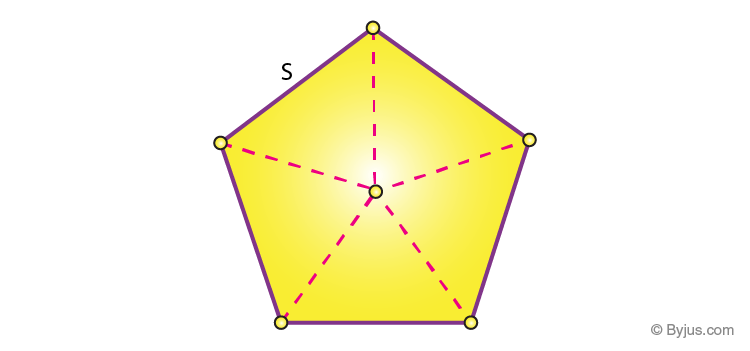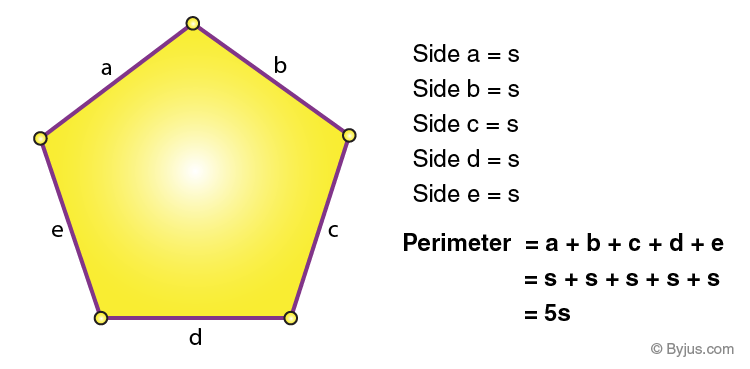# Area of Pentagon

A pentagon is a two-dimensional shape in geometry. A pentagon is a five-sided polygon, also called 5-gon. It can be regular as well as irregular. The sides and angles of a regular pentagon are equal with each. The interior angle equals to 108 degrees, and its exterior angle is equal to 72 degrees. Area of a pentagon is the region occupied by it in a plane and perimeter is the sum of all its sides.

The number of diagonals in a Pentagon is five.

Number of diagonals in any pentagon = {n*(n-3)}/2, where n = number of sides

In case of pentagon, the number of sides is equal to 5, n = 5.

Therefore, Number of diagonals of pentagon = [5(5-3)]/2 = (5 x 2)/2 = 5## Area of Pentagon Formula

Area of a pentagon, $$A = \frac{1}{4}\sqrt{5(5+2\sqrt{5})}s^{2}$$

This formula is for any pentagon, whether it is regular or irregular.

Area of regular pentagon can be found out in 2 ways.Area of Pentagon is given by 5/2 x s x a; where s is the side of the Pentagon, and a is the apothem length.

Apothem is the line from the center of the pentagon to a side, intersecting the side at 90 degrees right angle.

### Solved Examples

Example 1: Let’s take the pentagon with side length is 5 units and apothem length is 2 units

Area of pentagon is = 5/2 x s x a

= 5/2 x 5 x 2

=25 square centimetres.

Example 2: Find the area of a pentagon of side 5 cm and apothem length 3 cm?

Solution :

Given,

s = 5 cm

a = 3 cm

Area of a pentagon

= 37.5 cm

### How to Find Area of Pentagon?

II. Divide the regular pentagon into five equal triangles.Area of triangle is given by = ½ x base × height.

Area of Pentagon is given by = 5 × Area of a triangle.

## Perimeter of Pentagon

The perimeter of a pentagon is the total length of its boundaries. Therefore, it is equal to the sum of all its five sides. For a regular pentagon, the perimeter is given by:Example: If the side length of a regular pentagon is equal to 7 cm. Then find its perimeter.

Solution: Given, length of the side of pentagon = 7 cm

Perimeter of pentagon = 5 x side = 5 x 7 = 35 cms

## Properties of Pentagon

• A pentagon has five sides, five vertices and five diagonals.
• A regular pentagon has all its interior angles equal to 108 degrees
• A regular pentagon has all its exterior angles equal to 72 degrees
• Area of regular pentagon is equal to 1.7204774 × s2
• Sum of all the angles of regular or irregular pentagon is equal to 540 degrees